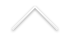Group theory is the study of symmetries. Roughly speaking, symmetry is an operation on a certain object or symmetries that preserve a particular pattern form an algebraic structure that can be thought as a group in some sense. More precisely, a group is a set of elements equipped with an operation that combines any two elements of the set to give a third element which also belongs to the set and satisfies three extra conditions called group axioms (associativity, identity and invertibility). The history of group theory, a mathematical domain studying groups in their various forms, has evolved in parallel threads. There are three historical roots of group theory: the theory of algebraic equations, number theory and geometry. Lagrange, Abel and Galois were early researchers in the field of group theory. On the other hand, while the concept of a ring was introduced by Dedekind in 1880, and the term 'ring' was coined by Hilbert in 1892, the first axiomatic definition of a ring was given by Adolf Fraenkel in 1914 and Emmy Noether gave the first axiomatic foundation of the theory of commutative rings in her monumental paper Ideal Theory in Rings in 1921.

Today group theory and ring theory are so deeply related that is difficult to find someone who works in only one of these major areas and here the interests are as varied as representation theory (of algebras and groups), algebraic geometry (theory of invariants, differential operators), arithmetic (orders, Brauer group), homology, functional analysis (algebra operators), Lie theory, geometry groups, K-theory, group algebras, varieties of algebras, identities (polynomial and groups), ...

Researchers:

• Guilherme Leal
• Ilir Snopche
• Severino Collier
• Luciane Quoos Conte
• Nicolas Puignau